# Search Results (43)

View
Selected filters:
• Ratios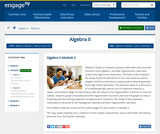Conditions of Use:
Remix and Share
Rating

Module 2 builds on students previous work with units and with functions from Algebra I, and with trigonometric ratios and circles from high school Geometry. The heart of the module is the study of precise definitions of sine and cosine (as well as tangent and the co-functions) using transformational geometry from high school Geometry. This precision leads to a discussion of a mathematically natural unit of rotational measure, a radian, and students begin to build fluency with the values of the trigonometric functions in terms of radians. Students graph sinusoidal and other trigonometric functions, and use the graphs to help in modeling and discovering properties of trigonometric functions. The study of the properties culminates in the proof of the Pythagorean identity and other trigonometric identities.

Subject:
Algebra
Material Type:
Module
Provider:
New York State Education Department
Provider Set:
EngageNY
08/15/2014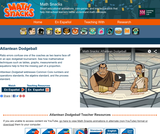Rating

Ratio errors confuse a dodgeball coach as two teams face off in an epic tournament. See how mathematical techniques such as tables, graphs, measurements and equations help to find the missing part of a proportion.

Subject:
Mathematics
Material Type:
Lecture
Provider:
Learning Games Lab
Author:
NMSU Learning Gams Lab
07/15/2015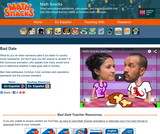Rating

True love has the right ratio. In this humorous animation, the number of words spoken by each partner predicts whether a date goes well or horribly. What do you do when someone asks if you listen to country music backwards, but won't let you get a word in edgewise?

Subject:
Mathematics
Material Type:
Lecture
Provider:
Learning Games Lab
Author:
NMSU Learning Games Lab
07/15/2015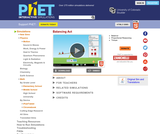Conditions of Use:
No Strings Attached
Rating

Play with objects on a teeter totter to learn about balance. Test what you've learned by trying the Balance Challenge game.

Subject:
Physics
Material Type:
Simulation
Provider:
University of Colorado Boulder
Provider Set:
PhET Interactive Simulations
Author:
Ariel Paul
John Blanco
Kathy Perkins
Michael Dubson
Trish Loeblein
04/24/2012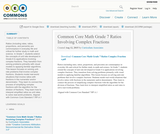Rating

Ratios (including rates, ratios, proportions, and percents) are commonplace in everyday life and critical for further study in math and science. In Grade 7, students extend the concepts of unit rate developed in Grade 6 to applications involving complex fractions. They transition from solving problems primarily with visual models to applying familiar algorithms. This lesson focuses on solving unit-rate problems that involve complex fractions. Students model real-world situations that involve ratios with fractions in the numerator and/or denominator. They learn to connect the process of simplifying complex fractions with the algorithm for the division of fractions. They learn how to interpret simplified ratios as unit rates to solve real-world problems.
Aligned with Common Core Standard 7.RP.A.1

Subject:
Mathematics
Material Type:
Lesson Plan
Author:
Curriculum Associates
01/28/2016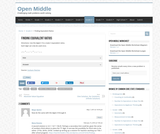Conditions of Use:
Remix and Share
Rating

Open middle problems require a higher depth of knowledge than most problems that assess procedural and conceptual understanding. They support the Common Core State Standards and provide students with opportunities for discussing their thinking.

The Finding Equivalent Ratios problem asks students to use the digits 1-9 to create 3 equivalent ratios made up of single and double digit numbers.

Subject:
Mathematics
Material Type:
Activity/Lab
Provider:
Open Middle
08/21/2017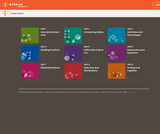Conditions of Use:
No Strings Attached
Rating

Student facing mathematics units. Covers area and surface area, ratios, dividing fractions, arithmetic in base ten, expressions and equations, rational numbers, and data sets/distributions.

Subject:
Mathematics
Material Type:
Unit of Study
Provider:
Open Up Resources
05/21/2019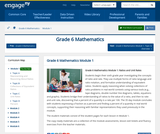Conditions of Use:
Remix and Share
Rating

Students begin their sixth grade year investigating the concepts of ratio and rate. They use multiple forms of ratio language and ratio notation, and formalize understanding of equivalent ratios. Students apply reasoning when solving collections of ratio problems in real world contexts using various tools (e.g., tape diagrams, double number line diagrams, tables, equations and graphs). Students bridge their understanding of ratios to the value of a ratio, and then to rate and unit rate, discovering that a percent of a quantity is a rate per 100. The 35 day module concludes with students expressing a fraction as a percent and finding a percent of a quantity in real world concepts, supporting their reasoning with familiar representations they used previously in the module.

Subject:
Ratios and Proportions
Material Type:
Module
Provider:
New York State Education Department
Provider Set:
EngageNY
05/14/2013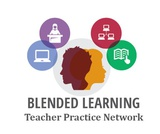Conditions of Use:
Remix and Share
Rating

In this problem-based learning module, students will be given the chance to plan their idea of the perfect party.  They are given a budget of \$2,500, this is the maximum amount of money they can use.  The goal is for students to plan a party that they think people would want to attend and would enjoy being a part of.  The students will need to come up with categories of what their party will need (food/drink, decorations, entertainment, location, etc).  These will then be the stations students will move at their own pace through to complete the party planning.  At each station they will need to identify what they are doing to have/do for the party and how much it will cost.  They will then have to figure out the unit cost (cost per person) for that category. The final station should allow for students to find the total cost of their part and total unit cost per person for the party.  If the total cost exceeds \$2,500 students should make adjustments as needed.Students will then create an advertisement (commercial, flyer, poster etc.) to promote their party as the “PARTY OF THE YEAR!”Students will then present these advertisements to school staff, parents, administrators etc. to vote on the party they would want to throw for their own child. They should take into consideration cost per person, entertainment, and enjoyment of the party.

Subject:
Mathematics
Material Type:
Lesson Plan
Author:
Blended Learning Teacher Practice NetworkConditions of Use:
Read the Fine Print
Rating

This lab exercise exposes students to a potentially new alternative energy source hydrogen gas. Student teams are given a hydrogen generator and an oxygen generator. They balance the chemical equation for the combustion of hydrogen gas in the presence of oxygen. Then they analyze what the equation really means. Two hypotheses are given, based on what one might predict upon analyzing the chemical equation. Once students have thought about the process, they are walked through the experiment and shown how to collect the gas in different ratios. By trial and error, students determine the ideal combustion ratio. For both volume of explosion and kick generated by explosion, they qualitatively record results on a 0-4 scale. Then, students evaluate their collected results to see if the hypotheses were correct and how their results match the theoretical equation. Students learn that while hydrogen will most commonly be used for fuel cells (no combustion situation), it has been used in rocket engines (for which a tremendous combustion occurs).

Subject:
Engineering
Chemistry
Material Type:
Activity/Lab
Provider:
TeachEngineering
Provider Set:
TeachEngineering
Author:
Courtney Herring
Stephen Dent
09/18/2014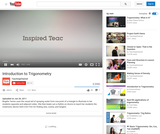Rating

Brigitte Tennis uses the visual aid of spraying water from one point of a triangle to illustrate to her students opposite and adjacent sides. She then beats out a rhythm on drums to teach her students the mnemonic device SOH-CAH-TOA for finding sine, cosine, and tangent.

Subject:
Education
Trigonometry
Material Type:
Lesson Plan
Teaching/Learning Strategy
Provider:
Teaching Channel
Provider Set:
Teaching Channel
Author:
Brigitte Tennis
11/02/2012Conditions of Use:
Remix and Share
Rating

Fractions and Decimals

Type of Unit: Concept

Prior Knowledge

Students should be able to:

Multiply and divide whole numbers and decimals.
Multiply a fraction by a whole number.
Multiply a fraction by another fraction.
Write fractions in equivalent forms, including converting between improper fractions and mixed numbers.
Understand the meaning and structure of decimal numbers.

Lesson Flow

This unit extends students’ learning from Grade 5 about operations with fractions and decimals.

The first lesson informally introduces the idea of dividing a fraction by a fraction. Students are challenged to figure out how many times a 14-cup measuring cup must be filled to measure the ingredients in a recipe. Students use a variety of methods, including adding 14 repeatedly until the sum is the desired amount, and drawing a model. In Lesson 2, students focus on dividing a fraction by a whole number. They make a model of the fraction—an area model, bar model, number line, or some other model—and then divide the model into whole numbers of groups. Students also work without a model by looking at the inverse relationship between division and multiplication. Students explore methods for dividing a whole number by a fraction in Lesson 3, for dividing a fraction by a unit fraction in Lesson 4, and for dividing a fraction by another fraction in Lesson 6. Students examine several methods and models for solving such problems, and use models to solve similar problems.

Students apply their learning to real-world contexts in Lesson 6 as they solve word problems that require dividing and multiplying mixed numbers. Lesson 7 is a Gallery lesson in which students choose from a number of problems that reinforce their learning from the previous lessons.

Students review the standard long-division algorithm for dividing whole numbers in Lesson 8. They discuss the different ways that an answer to a whole number division problem can be expressed (as a whole number plus a remainder, as a mixed number, or as a decimal). Students then solve a series of real-world problems that require the same whole number division operation, but have different answers because of how the remainder is interpreted.

Students focus on decimal operations in Lessons 9 and 10. In Lesson 9, they review addition, subtraction, multiplication, and division with decimals. They solve decimal problems using mental math, and then work on a card sort activity in which they must match problems with diagram and solution cards. In Lesson 10, students review the algorithms for the four basic decimal operations, and use estimation or other methods to place the decimal points in products and quotients. They solve multistep word problems involving decimal operations.

In Lesson 11, students explore whether multiplication always results in a greater number and whether division always results in a smaller number. They work on a Self Check problem in which they apply what they have learned to a real-world problem. Students consolidate their learning in Lesson 12 by critiquing and improving their work on the Self Check problem from the previous lesson. The unit ends with a second set of Gallery problems that students complete over two lessons.

Subject:
Mathematics
Ratios and Proportions
Material Type:
Unit of Study
Provider:
PearsonConditions of Use:
Remix and Share
Rating

Getting Started

Type of Unit: Introduction

Prior Knowledge

Students should be able to:

Solve and write numerical equations for whole number addition, subtraction, multiplication, and division problems.
Use parentheses to evaluate numerical expressions.
Identify and use the properties of operations.

Lesson Flow

In this unit, students are introduced to the rituals and routines that build a successful classroom math community and they are introduced to the basic features of the digital course that they will use throughout the year.

An introductory card sort activity matches students with their partner for the week. Then over the course of the week, students learn about the lesson routines: Opening, Work Time, Ways of Thinking, Apply the Learning, Summary of the Math, and Reflection. Students learn how to present their work to the class, the importance of taking responsibility for their own learning, and how to effectively participate in the classroom math community.

Students then work on Gallery problems to further explore the program’s technology resources and tools and learn how to organize their work.

The mathematical work of the unit focuses on numerical expressions, including card sort activities in which students identify equivalent expressions and match an expression card to a word card that describes its meaning. Students use the properties of operations to identify equivalent expressions and to find unknown values in equations.

Subject:
Mathematics
Material Type:
Unit of Study
Provider:
PearsonConditions of Use:
Remix and Share
Rating

Ratios

Type of Unit: Concept

Prior Knowledge

Students should be able to:

Calculate with whole numbers up to 100 using all four operations.
Understand fraction notation and percents and translate among fractions, decimal numbers, and percents.
Interpret and use a number line.
Use tables to solve problems.
Use tape diagrams to solve problems.
Sketch and interpret graphs.
Write and interpret equations.

Lesson Flow

The first part of the unit begins with an exploration activity that focuses on a ratio as a way to compare the amount of egg and the amount of flour in a mixture. The context motivates a specific understanding of the use of, and need for, ratios as a way of making comparisons between quantities. Following this lesson, the usefulness of ratios in comparing quantities is developed in more detail, including a contrast to using subtraction to find differences. Students learn to interpret and express ratios as fractions, as decimal numbers, in a:b form, in words, and as data; they also learn to identify equivalent ratios.

The focus of the middle part of the unit is on the tools used to represent ratio relationships and on simplifying and comparing ratios. Students learn to use tape diagrams first, then double number lines, and finally ratio tables and graphs. As these tools are introduced, students use them in problem-solving contexts to solve ratio problems, including an investigation of glide ratios. Students are asked to make connections and distinctions among these forms of representation throughout these lessons. Students also choose a ratio project in this part of the unit (Lesson 8).

The third and last part of the unit covers understanding percents, including those greater than 100%.

Students have ample opportunities to check, deepen, and apply their understanding of ratios, including percents, with the selection of problems in the Gallery.

Subject:
Mathematics
Statistics and Probability
Material Type:
Unit of Study
Provider:
Pearson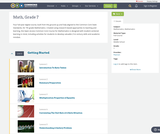Conditions of Use:
Remix and Share
Rating

Four full-year digital course, built from the ground up and fully-aligned to the Common Core State Standards, for 7th grade Mathematics. Created using research-based approaches to teaching and learning, the Open Access Common Core Course for Mathematics is designed with student-centered learning in mind, including activities for students to develop valuable 21st century skills and academic mindset.

Subject:
Mathematics
Material Type:
Full Course
Provider:
Pearson
10/06/2016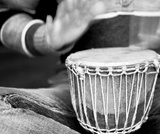Conditions of Use:
Remix and Share
Rating

Getting Started

Type of Unit: Introduction

Prior Knowledge

Students should be able to:

Understand ratio concepts and use ratios.
Use ratio and rate reasoning to solve real-world problems.
Identify and use the multiplication property of equality.

Lesson Flow

This unit introduces students to the routines that build a successful classroom math community, and it introduces the basic features of the digital course that students will use throughout the year.

An introductory card sort activity matches students with their partner for the week. Then over the course of the week, students learn about the routines of Opening, Work Time, Ways of Thinking, Apply the Learning (some lessons), Summary of the Math, Reflection, and Exercises. Students learn how to present their work to the class, the importance of students’ taking responsibility for their own learning, and how to effectively participate in the classroom math community.

Students then work on Gallery problems, to further explore the resources and tools and to learn how to organize their work.

The mathematical work of the unit focuses on ratios and rates, including card sort activities in which students identify equivalent ratios and match different representations of an equivalent ratio. Students use the multiplication property of equality to justify solutions to real-world ratio problems.

Subject:
Mathematics
Material Type:
Unit of Study
Provider:
PearsonConditions of Use:
Remix and Share
Rating

Discuss the important ways that listeners contribute to mathematical discussions during Ways of Thinking presentations. Then use ratio and rate reasoning to solve a problem about ingredients in a stew.Key ConceptsStudents find the unit rate of a ratio situation.Goals and Learning ObjectivesContribute as listeners during the Ways of Thinking discussion.Understand the concept of a unit rate that is associated with a ratio.Use rate reasoning to solve real-world problems.

Subject:
Ratios and Proportions
Material Type:
Lesson Plan
Provider:
PearsonConditions of Use:
Remix and Share
Rating

Students use ratio cards to find equivalencies and form partnerships for the week. As a class, students discuss and decide on classroom norms.Give each student a ratio card. Instruct students to find a classmate whose card has a ratio that is equivalent to theirs. Classmates with equivalent ratios are now partners for the week. With the class, discuss and decide on classroom norms, or rules. Tell students how to access the application they will use this year.Key ConceptsStudents understand that ratio relationships are multiplicative. They use ratio tables to show ratio relationships.Goals and Learning ObjectivesDistinguish between ratio tables and tables that do no show equivalent ratios.Understand how ratio tables are used to solve ratio problems.Use the basic features of the application.Create and understand the classroom norms.Use mathematical reasoning to justify an answer.

Subject:
Ratios and Proportions
Material Type:
Lesson Plan
Provider:
PearsonConditions of Use:
Remix and Share
Rating

Review the ways classroom habits and routines can strengthen students’ mathematical character. Explain what a Gallery is and how to choose a Gallery problem to solve. Direct students to choose one of three Gallery problems that introduce the unit’s technology resources. The three Gallery problems combine working with ratios and rates with the application resources available with this unit.Key ConceptsStudents understand that a Gallery gives them a choice of problems to solve. Students think about the features of the problems to use when choosing a problem. Students know how to work on a Gallery problem and present a solution.Goals and Learning ObjectivesKnow how to choose a problem from a Gallery.

Subject:
Ratios and Proportions
Material Type:
Lesson Plan
Provider:
PearsonConditions of Use:
Remix and Share
Rating

Proportional Relationships

Type of Unit: Concept

Prior Knowledge

Students should be able to:

Understand what a rate and ratio are.
Make a ratio table.
Make a graph using values from a ratio table.

Lesson Flow

Students start the unit by predicting what will happen in certain situations. They intuitively discover they can predict the situations that are proportional and might have a hard time predicting the ones that are not. In Lessons 2–4, students use the same three situations to explore proportional relationships. Two of the relationships are proportional and one is not. They look at these situations in tables, equations, and graphs. After Lesson 4, students realize a proportional relationship is represented on a graph as a straight line that passes through the origin. In Lesson 5, they look at straight lines that do not represent a proportional relationship. Lesson 6 focuses on the idea of how a proportion that they solved in sixth grade relates to a proportional relationship. They follow that by looking at rates expressed as fractions, finding the unit rate (the constant of proportionality), and then using the constant of proportionality to solve a problem. In Lesson 8, students fine-tune their definition of proportional relationship by looking at situations and determining if they represent proportional relationships and justifying their reasoning. They then apply what they have learned to a situation about flags and stars and extend that thinking to comparing two prices—examining the equations and the graphs. The Putting It Together lesson has them solve two problems and then critique other student work.

Gallery 1 provides students with additional proportional relationship problems.

The second part of the unit works with percents. First, percents are tied to proportional relationships, and then students examine percent situations as formulas, graphs, and tables. They then move to a new context—salary increase—and see the similarities with sales taxes. Next, students explore percent decrease, and then they analyze inaccurate statements involving percents, explaining why the statements are incorrect. Students end this sequence of lessons with a formative assessment that focuses on percent increase and percent decrease and ties it to decimals.

Students have ample opportunities to check, deepen, and apply their understanding of proportional relationships, including percents, with the selection of problems in Gallery 2.

Subject:
Mathematics
Ratios and Proportions
Material Type:
Unit of Study
Provider:
PearsonConditions of Use:
Remix and Share
Rating

Samples and ProbabilityType of Unit: ConceptualPrior KnowledgeStudents should be able to:Understand the concept of a ratio.Write ratios as percents.Describe data using measures of center.Display and interpret data in dot plots, histograms, and box plots.Lesson FlowStudents begin to think about probability by considering the relative likelihood of familiar events on the continuum between impossible and certain. Students begin to formalize this understanding of probability. They are introduced to the concept of probability as a measure of likelihood, and how to calculate probability of equally likely events using a ratio. The terms (impossible, certain, etc.) are given numerical values. Next, students compare expected results to actual results by calculating the probability of an event and conducting an experiment. Students explore the probability of outcomes that are not equally likely. They collect data to estimate the experimental probabilities. They use ratio and proportion to predict results for a large number of trials. Students learn about compound events. They use tree diagrams, tables, and systematic lists as tools to find the sample space. They determine the theoretical probability of first independent, and then dependent events. In Lesson 10 students identify a question to investigate for a unit project and submit a proposal. They then complete a Self Check. In Lesson 11, students review the results of the Self Check, solve a related problem, and take a Quiz.Students are introduced to the concept of sampling as a method of determining characteristics of a population. They consider how a sample can be random or biased, and think about methods for randomly sampling a population to ensure that it is representative. In Lesson 13, students collect and analyze data for their unit project. Students begin to apply their knowledge of statistics learned in sixth grade. They determine the typical class score from a sample of the population, and reason about the representativeness of the sample. Then, students begin to develop intuition about appropriate sample size by conducting an experiment. They compare different sample sizes, and decide whether increasing the sample size improves the results. In Lesson 16 and Lesson 17, students compare two data sets using any tools they wish. Students will be reminded of Mean Average Deviation (MAD), which will be a useful tool in this situation. Students complete another Self Check, review the results of their Self Check, and solve additional problems. The unit ends with three days for students to work on Gallery problems, possibly using one of the days to complete their project or get help on their project if needed, two days for students to present their unit projects to the class, and one day for the End of Unit Assessment.

Subject:
Mathematics
Statistics and Probability
Material Type:
Unit of Study
Provider:
PearsonConditions of Use:
Remix and Share
Rating

Students begin to formalize their understanding of probability. They are introduced to the concept of probability as a measure of likelihood and how to calculate probability as a ratio. The terms discussed (impossible, certain, etc.) in Lesson 1 are given numerical values.Key ConceptsStudents will think of probability as a ratio; it can be written as a fraction, decimal, or a percent ranging from 0 to 1.Students will think about ratio and proportion to predict results.Goals and Learning ObjectivesDefine probability as a measure of likelihood and the ratio of favorable outcomes to the total number of outcomes for an event.Predict results based on theoretical probability using ratio and proportion.

Subject:
Statistics and Probability
Material Type:
Lesson Plan
Provider:
Pearson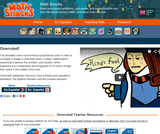Rating

Two besotted rulers must embrace proportional units in order to unite their lands. It takes mathematical reasoning to identify the problem, and solution, when engineers from Queentopia and Kingopolis build a bridge to meet in the middle of the river.

Subject:
Mathematics
Material Type:
Lecture
Provider:
Learning Games Lab
Author:
NMSU Learning Games Lab
07/15/2015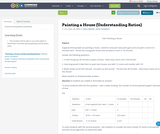Rating

A task involving Ratios and Rates

Subject:
Mathematics
Material Type:
Activity/Lab
Author:
Elana Webster
James Strickland
01/28/2016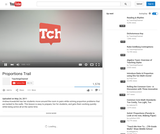Rating

Andrea Kowalchik has her students move around the room in pairs while solving proportion problems that are tacked to the walls. This lesson is easy to prepare, fun for students, and gets them working quickly while being active all at the same time.

Subject:
Education
Ratios and Proportions
Material Type:
Lesson Plan
Teaching/Learning Strategy
Provider:
Teaching Channel
Provider Set:
Teaching Channel
Author:
Andrea Kowalchik
11/02/2012Rating

In this activity students view and analyze images of ramps and steps to see if they conform to the requirements of the Americans with Disabilities Act. Students measure horizontal and vertical distances and compute the percent slope for each image. Image measurements are performed using WebImage, a Web-based, customized version of ImageJ.

Subject:
Education
Mathematics
Astronomy
Material Type:
Activity/Lab
Diagram/Illustration
Interactive
Simulation
Unit of Study
Provider:
UCAR Staff
Provider Set:
Simplified Image Management and Processing Learning Environment for Science (SIMPLE Science)
11/02/2014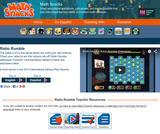Rating

The battle is on in this game where you build your own potions! Check your ratios to win this mixture mix-off. Ratio Rumble guides students in: identifying ratios when used in a variety of contextual situations; providing visual representations of ratios; solving common problems or communicating by using rate, particularly unit rates; and explaining why ratios and rates naturally relate to fractions and decimals.

Subject:
Mathematics
Material Type:
Game
Interactive
Provider:
Learning Games Lab
Author:
NMSU Learning Games Lab
07/20/2015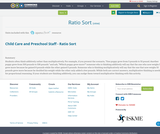Conditions of Use:
Remix and Share
Rating

Students often think additively rather than multiplicatively. For example, if you present the scenario, "One puppy grew from 5 pounds to 10 pound. Another puppy grew from 100 pounds to 108 pounds." and ask, "Which puppy grew more?" someone who is thinking additively will say that the one who now weighs 108 grew more because he gained 8 pounds while the other gained 5 pounds. Someone who is thinking multiplicatively will say that the one that now weighs 10 pounds grew more because he doubled his weight while the other only added a few pounds. While both are correct answers, multiplicative thinking is needed for proportional reasoning. If your students are thinking additively, you can nudge them toward multiplicative thinking with this activity.

Subject:
Mathematics
Material Type:
Activity/Lab
Interactive
Lesson Plan
Author:
Connie Rivera
11/07/2017Conditions of Use:
No Strings Attached
Rating

This module covers the addition and subtraction of rational expressions.

Subject:
Algebra
Material Type:
Provider:
Rice University
Provider Set:
Connexions
Author:
Kenny Felder
02/16/2011Conditions of Use:
No Strings Attached
Rating

This module covers the division of polynomials as rational expressions.

Subject:
Algebra
Material Type:
Provider:
Rice University
Provider Set:
Connexions
Author:
Kenny Felder
02/16/2011Conditions of Use:
No Strings Attached
Rating

This module covers dividing polynomials as rational expressions.

Subject:
Algebra
Material Type:
Provider:
Rice University
Provider Set:
Connexions
Author:
Kenny Felder
02/16/2011Conditions of Use:
No Strings Attached
Rating

This module provides an introduction to rational expressions.

Subject:
Algebra
Material Type:
Provider:
Rice University
Provider Set:
Connexions
Author:
Kenny Felder
02/16/2011Conditions of Use:
No Strings Attached
Rating

This module introduces rational expressions in equations.

Subject:
Algebra
Material Type:
Provider:
Rice University
Provider Set:
Connexions
Author:
Kenny Felder
02/16/2011Conditions of Use:
No Strings Attached
Rating

Algebraic division of polynomials.

Subject:
Algebra
Material Type:
Provider:
Rice University
Provider Set:
Connexions
Author:
Kenny Felder
02/16/2011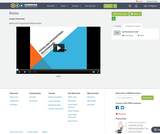Conditions of Use:
Remix and Share
Rating

Ratios and Proportional Relationshipsratios

Subject:
Mathematics
Material Type:
Lesson Plan
Author:
sameano porchea
06/13/2017
Remix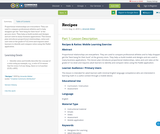Conditions of Use:
No Strings Attached
Rating

Proportional relationships are everywhere. They are used to compare professional athletes and to help shoppers get the “best bang for their buck” at the grocery store. They help us build models and designs and are used in many business applications. This lesson plan introduces proportional relationships, ratios and unit rates at the grade 6/7 (C) level and requires adult learners to identify and compare ratios using the Padlet application.

Subject:
Mathematics
Material Type:
Assessment
Lesson
Author:
Amanda Weber
08/08/2019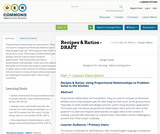Conditions of Use:
No Strings Attached
Rating

Proportional relationships are everywhere. They are used to compare professional athletes and to help shoppers get the “best bang for their buck” at the grocery store. They help us build models and designs and are used in many business applications. This lesson plan introduces proportional relationships, ratios and unit rates at the grade 6/7 (C) level and requires adult learners to apply ratios in the context of cooking. Learners will need learn to research basic information and be challenged to present their “final recipe” creatively.

Subject:
English Language Arts
Mathematics
Material Type:
Lesson
Author:
Carolyn Wilson
01/18/2017
Remix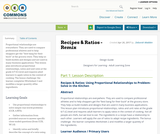Conditions of Use:
Remix and Share
Rating

Proportional relationships are everywhere. They are used to compare professional athletes and to help shoppers get the “best bang for their buck” at the grocery store. They help us build models and designs and are used in many business applications. This lesson plan introduces proportional relationships, ratios and unit rates at the grade 6/7 (C) level and requires adult learners to apply ratios in the context of cooking. The bonus challenge: the learner completes Worksheet 2 and modifies a larger quantity ofthe ingredients.

Subject:
English Language Arts
Mathematics
Material Type:
Homework/Assignment
Lesson
Author:
08/08/2019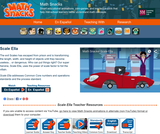Rating

The evil Scaleo has escaped from prison and is transforming the length, width, and height of objects until they become useless – or dangerous. Who can put things right? Superheroine Scale Ella uses the power of scale factor to foil the villain.

Subject:
Mathematics
Material Type:
Lecture
Provider:
Learning Games Lab
Author:
NMSU Learning Games Lab
07/15/2015Conditions of Use:
Read the Fine Print
Rating

Students learn how different characteristics of shapes—side lengths, perimeter and area—change when the shapes are scaled, either enlarged or reduced. Student pairs conduct a “scaling investigation” to measure and calculate shape dimensions (rectangle, quarter circle, triangle; lengths, perimeters, areas) from a bedroom floorplan provided at three scales. They analyze their data to notice the mathematical relationships that hold true during the scaling process. They see how this can be useful in real-world situations like when engineers design wearable or implantable biosensors. This prepares students for the associated activity in which they use this knowledge to help them reduce or enlarge their drawings as part of the process of designing their own wearables products. Pre/post-activity quizzes, a worksheet and wrap-up concepts handout are provided.

Subject:
Career and Technical Education
Mathematics
Measurement and Data
Numbers and Operations
Material Type:
Lesson
Provider:
TeachEngineering
Author:
Denise W. Carlson
Evelynne Pyne
Lauchlin Blue